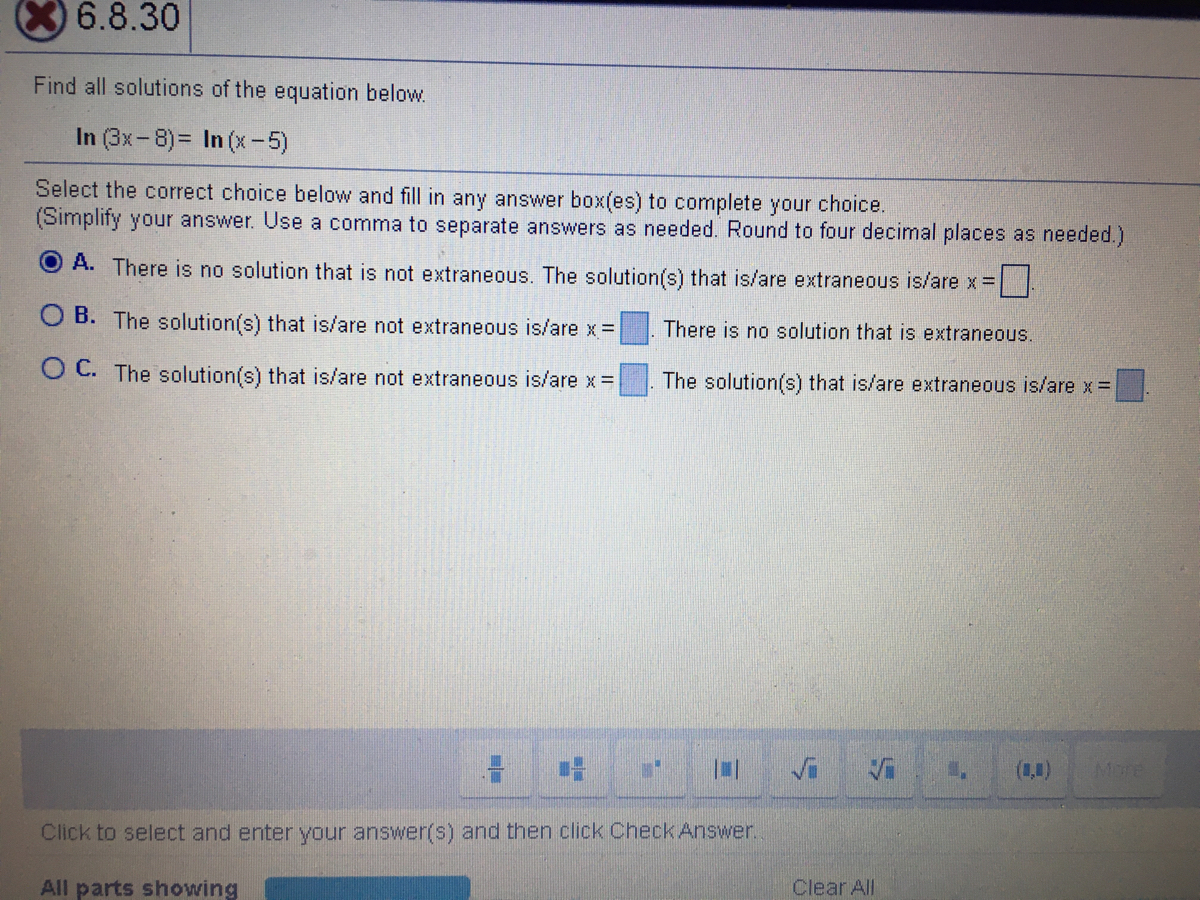# Find all solutions of the equation below. In (3x-8)= In (x-5) Select the correct choice below and fill in any answer box(es) to complete your choice. (Simplify your answer. Use a comma to separate answers as needed. Round to four decimal places as needed.) O A. There is no solution that is not extraneous. The solution(s) that is/are extraneous is/are x = O B. The solution(s) that is/are not extraneous is/are x = There is no solution that is extraneous. O C. The solution(s) that is/are not extraneous is/are x = The solution(s) that is/are extraneous is/are x =

Question
Find all solutions to this equationhelp_outlineImage TranscriptioncloseFind all solutions of the equation below. In (3x-8)= In (x-5) Select the correct choice below and fill in any answer box(es) to complete your choice. (Simplify your answer. Use a comma to separate answers as needed. Round to four decimal places as needed.) O A. There is no solution that is not extraneous. The solution(s) that is/are extraneous is/are x = O B. The solution(s) that is/are not extraneous is/are x = There is no solution that is extraneous. O C. The solution(s) that is/are not extraneous is/are x = The solution(s) that is/are extraneous is/are x = fullscreen

### Want to see this answer and more?

Experts are waiting 24/7 to provide step-by-step solutions in as fast as 30 minutes!*

*Response times may vary by subject and question complexity. Median response time is 34 minutes for paid subscribers and may be longer for promotional offers.
Tagged in
Math
Algebra

### Other